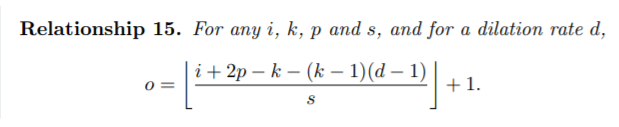# Converting tensorflow model to pytorch: issue with padding

I was re-writing a model in pytorch from tensorflow and came across of issues with padding. In the tensorflow model, the padding used in “SAME” and I tried to replicate that according to this:After loading the tensorflow weights in, everything looks good until I hit the layers with “SAME” padding. I’ve read some previous post where others have had some trouble with replicating “SAME” padding in pytorch, but I haven’t come across posts where this was a problem when converting a tensorflow model to a pytorch model. The documentation on how “SAME” padding is calculated also didn’t seem to be very detailed (but maybe I’m looking at the wrong place).

in_channel = 526
out_channel = 64
input_shape = 140x140
kernel_size = 3x3
dilation = 1
stride = 2

Any advice or suggestions here would greatly help!

Hi,

PyTorch does not support `same` padding explicitly, but you can obviously find the proper padding size `p` using the exact same formula you have provided. In your case, something especial is that padding size cannot be an integer number which means you have to have one side more padded.

``````(2*(output-1) - input - kernel)*(1 / stride)
``````

Will give you 70.5 as the proper padding size. So, symmetric padding is not possible but for asymmetric case you can achieve it using `torch.nn.functional.pad(input, (int, int, int, int))` for `left, right, top and bottom respectively.
Here is an example with your values:

``````x = torch.randn(1, 526, 140, 140)
x = F.pad(x, (71, 70, 71, 70), )
x = nn.Conv2d(526, 64, 3, 2)(x)
x.shape
``````

Bests

3 Likes

A note for anyone else who might go looking for the source of the formula referenced by the O.P. It is from A guide to convolution arithmetic for deep learning with accompanying repository at https://github.com/vdumoulin/conv_arithmetic